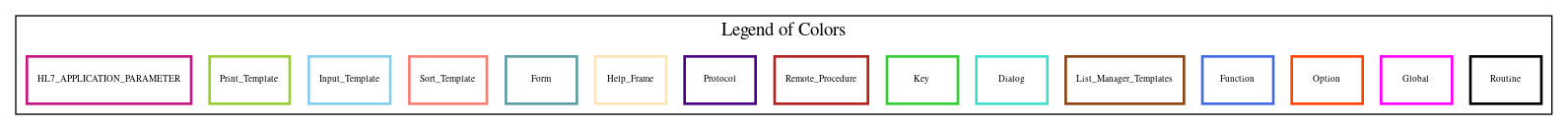Home   Package List   Routine Alphabetical List   Global Alphabetical List   FileMan Files List   FileMan Sub-Files List   Package Component Lists   Package-Namespace Mapping
Routine: PRCHDAM6

# Routine: PRCHDAM6

## Information

PRCHDAM6 ;WISC/DJM-PRINT AMENDMENT,ROUTINE 65 ;6/3/94 8:46 AM

## Source Information

Source file <PRCHDAM6.m>

## Call Graph### Call Graph Total: 1

Package Total Call Graph
IFCAP 1 (DATA,LCNT,LCNT1,LINE)^PRCHDAM4

## Caller Graph

### Legends:#### Package Component Superscript legend

 action A extended action Ea event driver Ed subscriber Su protocol O limited protocol LP run routine RR broker B edit E server Se print P screenman SM inquire I### Caller Graph Total: 1

Package Total Caller Graph
IFCAP 1 PRCHDAM1

## Entry Points

V ;;5.1;IFCAP;;Oct 20, 2000
E37 ;ITEM DISCOUNT Delete PRINT

## External References

Name Field # of Occurrence
DATA^PRCHDAM4 E36+23, E36+24, E36+27, E36+28, E36+30, E36+32, E37+10, E37+11
LCNT^PRCHDAM4 E36+2, E37+2
LCNT1^PRCHDAM4 E36+34, E37+11
LINE^PRCHDAM4 E36+20, E37+9

## Global Variables Directly Accessed

Name Line Occurrences  (* Changed,  ! Killed)
^PRC(443.6 - [#443.6] E36+3, E36+4, E36+5, E36+6, E36+12, E36+17, E36+18, E36+19, E37+3, E37+4
E37+5, E37+7, E37+8

## Local Variables

### Legend:

 >> Not killed explicitly * Changed ! Killed ~ Newed

Name Field # of Occurrence
>> AMEND E36+3, E36+4, E37+3, E37+4
CHANGE E36+1~, E36+4*, E36+5, E36+6, E37+4*, E37+5
CHANGES E36+1~, E36+5*, E36+11, E37+5*, E37+6
DATA E36+1~, E36+23*, E36+24*, E36+27*, E36+28*, E36+30*, E36+32*, E37+10*, E37+11*
DIS E36+1~, E37+8*, E37+11
DIS1 E36+1~
DIS2 E36+1~, E36+7!, E36+8*, E36+21*, E36+27, E36+32
FIELD E36+1~, E36+2*, E36+3*, E36+4, E36+8, E36+9, E36+17, E37+2*, E37+3*, E37+4
FLAGDISC E36+1~, E36+10*, E36+23
ITEMD E36+1~, E36+2!, E36+11*, E36+12, E36+17, E36+19, E37+6*, E37+7, E37+8
ITEMD( E36+11*
ITEMD0 E36+1~, E36+12*, E37+7*
LCNT E36+1~, E36+2, E36+20, E36+23, E36+24, E36+27, E36+28, E36+30, E36+32, E36+34
E37+2, E37+9, E37+10, E37+11
NEW E36+1~, E36+19*
NEWI E36+1~, E36+19*, E36+24, E36+28, E36+30, E36+32
NEWP E36+1~, E36+19*, E36+24, E36+28, E36+30
OLD E36+1~, E36+6*, E36+8, E36+9, E36+10, E37+5*, E37+11
PCT E36+1~, E36+17*, E36+18
PCT1 E36+1~, E36+17*
PCT2 E36+1~, E36+7!, E36+9*, E36+18*, E36+21*, E36+27, E36+30
>> PRCHAM E36+3, E36+4, E36+5, E36+6, E36+17, E36+18, E37+3, E37+4, E37+5
>> PRCHPO E36+3, E36+4, E36+5, E36+6, E36+12, E36+17, E36+18, E36+19, E37+3, E37+4
E37+5, E37+7, E37+8
U E36+11, E36+17, E36+19, E37+6, E37+8